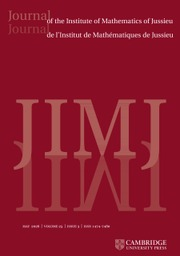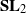Home
Hostname: page-component-cf9d5c678-dkwk2 Total loading time: 0.222 Render date: 2021-07-30T15:16:39.881Z Has data issue: true Feature Flags: { "shouldUseShareProductTool": true, "shouldUseHypothesis": true, "isUnsiloEnabled": true, "metricsAbstractViews": false, "figures": true, "newCiteModal": false, "newCitedByModal": true, "newEcommerce": true, "newUsageEvents": true }Journal of the Institute of Mathematics of Jussieu

# Extensions of differential representations of SL2 and tori

Published online by Cambridge University Press:  16 May 2012

Corresponding

## Abstract

Linear differential algebraic groups (LDAGs) measure differential algebraic dependencies among solutions of linear differential and difference equations with parameters, for which LDAGs are Galois groups. Differential representation theory is a key to developing algorithms computing these groups. In the rational representation theory of algebraic groups, one starts withand tori to develop the rest of the theory. In this paper, we give an explicit description of differential representations of tori and differential extensions of irreducible representation of. In these extensions, the two irreducible representations can be non-isomorphic. This is in contrast to differential representations of tori, which turn out to be direct sums of isotypic representations.

Type
Research Article
Information

## Access options

Get access to the full version of this content by using one of the access options below. (Log in options will check for institutional or personal access. Content may require purchase if you do not have access.)

## References

1.Bernstein, J. , Representations of-adic groups. Lectures by Joseph Bernstein. Written by Karl E. Rumelhart (Harvard University, Fall, 1992) (URL http://www.math.tau.ac.il/~bernstei/Publication_list/publication_texts/Bernst_Lecture_p-adic_repr.pdf).Google Scholar
2.Borel, A. , Linear algebraic groups, 2nd enlarged edn (Springer, 1991).CrossRefGoogle Scholar
3.Buium, A. , Geometry of differential polynomial functions. I. Algebraic groups, Amer. J. Math. 115 (6) (1993), 13851444.CrossRefGoogle Scholar
4.Cassidy, P. , Differential algebraic groups, Amer. J. Math. 94 (3) (1972), 891954.CrossRefGoogle Scholar
5.Cassidy, P. , The differential rational representation algebra on a linear differential algebraic group, J. Algebra 37 (2) (1975), 223238.CrossRefGoogle Scholar
6.Cassidy, P. , The classification of the semisimple differential algebraic groups and linear semisimple differential algebraic Lie algebras, J. Algebra 121 (1) (1989), 169238.CrossRefGoogle Scholar
7.Cassidy, P. and Singer, M. , Galois theory of parameterized differential equations and linear differential algebraic groups, in Differential equations and quantum groups, IRMA Lectures in Mathematics and Theoretical Physics, Volume 9. pp. 113155. (European Mathematical Society, Zürich, 2007).Google Scholar
8.Cassidy, P. and Singer, M. , A Jordan–Hölder theorem for differential algebraic groups, J. Algebra 328 (1) (2011), 190217.CrossRefGoogle Scholar
9.Cox, D., Little, J. and O’Shea, D. , Ideals, varieties, and algorithms, 3rd edn (Springer, 2007).CrossRefGoogle Scholar
10.Crumley, M. , Ultraproducts of Tannakian categories and generic representation theory of unipotent algebraic groups, PhD thesis, University of Toledo (URL http://arxiv.org/abs/1011.0460; 2010).Google Scholar
11.Di Vizio, L. and Hardouin, C. , Algebraic and differential generic Galois groups for-difference equations, followed by the appendix ‘The Galois-groupoid of a-difference system’ by Anne Granier (URL http://arxiv.org/abs/1002.4839; 2011).Google Scholar
12.Dreyfus, T. , The Kovacic’s algorithm for parameterized differential Galois theory (URL http://arxiv.org/abs/1110.1053; 2012).Google Scholar
13.Gillet, H., Gorchinskiy, S. and Ovchinnikov, A. , Parameterized Picard–Vessiot extensions and Atiyah extensions (URL http://arxiv.org/abs/1110.3526; 2012).Google Scholar
14.Hardouin, C. , Hypertranscendance des systèmes aux différences diagonaux, Compositio Math. 144 (3) (2008), 565581.CrossRefGoogle Scholar
15.Hardouin, C., Minchenko, A. and Ovchinnikov, A. , Characterization of differential reductivity and interpretation of the Stokes group of a-difference system as the unipotent radical (2012), in preparation.Google Scholar
16.Hardouin, C. and Singer, M. , Differential Galois theory of linear difference equations, Math. Ann. 342 (2) (2008), 333377.CrossRefGoogle Scholar
17.van Hoeij, M., Ragot, J. F., Ulmer, F. and Weil, J. A. , Liouvillian solutions of linear differential equations of order three and higher, J. Symbolic Comput. 28 (4–5) (1999), 589610.CrossRefGoogle Scholar
18.Hubert, E. , Essential components of an algebraic differential equation, J. Symbolic Comput. 28 (4–5) (1999), 657680.CrossRefGoogle Scholar
19.Humphreys, J. E. , Introduction to Lie algebras and representation theory. (Springer, Berlin, 1972).CrossRefGoogle Scholar
20.Kolchin, E. R. , Differential algebra and algebraic groups. (Academic Press, New York, 1973).Google Scholar
21.Kolchin, E. R. , Differential algebraic groups. (Academic Press, New York, 1985).Google Scholar
22.Kovacic, J. , An algorithm for solving second order linear homogeneous differential equations, J. Symbolic Comput. 2 (1) (1986), 343.CrossRefGoogle Scholar
23.Minchenko, A. and Ovchinnikov, A. , Zariski closures of reductive linear differential algebraic groups, Adv. Math. 227 (3) (2011), 11951224.CrossRefGoogle Scholar
24.Mustaţă, M. , Jet schemes of locally complete intersection canonical singularities, Invent. Math. 145 (3) (2001), 397424.CrossRefGoogle Scholar
25.Ovchinnikov, A. , Tannakian approach to linear differential algebraic groups, Transform. Groups 13 (2) (2008), 413446.CrossRefGoogle Scholar
26.Ovchinnikov, A. , Tannakian categories, linear differential algebraic groups, and parametrized linear differential equations, Transform. Groups 14 (1) (2009), 195223.CrossRefGoogle Scholar
27.van der Put, M. and Singer, M. F. , Galois theory of linear differential equations. (Springer, Berlin, 2003).CrossRefGoogle Scholar
28.Singer, M. F. and Ulmer, F. , Galois groups of second and third order linear differential equations, J. Symbolic Comput. 16 (3) (1993), 936.CrossRefGoogle Scholar
29.Singer, M. F. and Ulmer, F. , Liouvillian and algebraic solutions of second and third order linear differential equations, J. Symbolic Comput. 16 (3) (1993), 3773.CrossRefGoogle Scholar
30.Singer, M. F. and Ulmer, F. , Necessary conditions for Liouvillian solutions of (third order) linear differential equations, Appl. Algebra Engng, Comm. Comput. 6 (1) (1995), 122.CrossRefGoogle Scholar
31.Sit, W. , Differential algebraic subgroups ofand strong normality in simple extensions, Amer. J. Math. 97 (3) (1975), 627698.CrossRefGoogle Scholar
32.Springer, T. A. , Invariant theory. (Springer, Berlin, New York, 1977).CrossRefGoogle Scholar
33.Trushin, D. , Splitting fields and general differential Galois theory, Sb. Math. 201 (9) (2010), 13231353.CrossRefGoogle Scholar
34.Ulmer, F. and Weil, J. A. , A note on Kovacic’s algorithm, J. Symbolic Comput. 22 (2) (1996), 179200.CrossRefGoogle Scholar
35.Waterhouse, W. , Introduction to affine group schemes. (Springer, Berlin, 1979).CrossRefGoogle Scholar
36.Zobnin, A. , Essential properties of admissible orderings and rankings, in Contributions to general algebra 14, pp. 205221. (Verlag Johannes Heyn, 2004).Google Scholar
6
Cited by

# Send article to Kindle

Note you can select to send to either the @free.kindle.com or @kindle.com variations. ‘@free.kindle.com’ emails are free but can only be sent to your device when it is connected to wi-fi. ‘@kindle.com’ emails can be delivered even when you are not connected to wi-fi, but note that service fees apply.

Find out more about the Kindle Personal Document Service.

Extensions of differential representations of SL2 and tori
Available formats
×

# Send article to Dropbox

To send this article to your Dropbox account, please select one or more formats and confirm that you agree to abide by our usage policies. If this is the first time you use this feature, you will be asked to authorise Cambridge Core to connect with your <service> account. Find out more about sending content to Dropbox.

Extensions of differential representations of SL2 and tori
Available formats
×

# Send article to Google Drive

To send this article to your Google Drive account, please select one or more formats and confirm that you agree to abide by our usage policies. If this is the first time you use this feature, you will be asked to authorise Cambridge Core to connect with your <service> account. Find out more about sending content to Google Drive.

Extensions of differential representations of SL2 and tori
Available formats
×
×